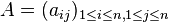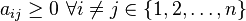# Metzler matrix

(diff) ← Older revision | Latest revision (diff) | Newer revision → (diff)
This article defines a property that can be evaluated for a square matrix with entries over the field of real numbers. In other words, given a square matrix (a matrix with an equal number of rows and columns) with entries over the field of real numbers, the matrix either satisfies or does not satisfy the property.
View other properties of square matrices with entries over the field of real numbers | View other properties of square matrices

## Definition

### Verbal definition

A square matrix is termed a Metzler matrix', quasipositive matrix, quasi-positive matrix, or essentially nonnegative matrix if all its off-diagonal entries are nonnegative.

### Algebraic description

Supposeis a positive integer. Asquare matrixwith real entries is termed a Metzler matrix', quasipositive matrix, quasi-positive matrix, or essentially nonnegative matrix if it satisfies the following condition:### Other equivalent descriptions

A square matrix with real entries is a Metzler matrix if and only if it is the negative of a Z-matrix. This can be used as an alternative definition.